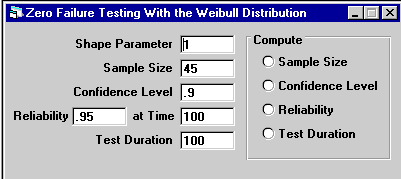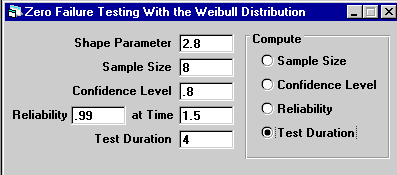Example 4
Home

Life Data Analysis

A new product is required to demonstrate 99% reliability at 1.5 million cycles with 80% confidence.  Given a Weibull shape parameter of 2.8, how long must 8 units be tested to demonstrate the required reliability assuming no failures?

### Solution

1. Select "Testing" from the menu, and the following screen appears.2. Enter the shape parameter of 2.8, a sample size of 8, a confidence level of 0.8, reliability of 0.99 and a time of 1.5.  Click the "Test Duration" radio button, and the test duration will be computed.  To demonstrate 99% reliability with 80% confidence at 1.5 million cycles requires testing 8 units for 4 million cycles with no failures.  This is shown in the figure below.3. Change the Test Duration to 1.5, and click the "Sample Size" radio button.   To demonstrate 99% reliability with 80% confidence at 1.5 million cycles requires testing 161 units for 1.5 million cycles with no failures.  By testing a sample size of 8 for 4 million cycles ( a total of 32 million test cycles) instead of 161 samples for 1.5 million cycles ( a total of 241.5 million test cycles), the total number of test cycles is reduces by 80%.  If the shape parameter is greater than 1, the total number of test cycles decreases as the test duration increases.